### Home > MC1 > Chapter 6 > Lesson 6.2.3 > Problem6-70

6-70.
1. . Write each of the mixed numbers below as a fraction greater than one, and write each the fractions greater than one as a mixed number. Include a diagram to explain each answer. Homework Help ✎

1. 4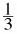2.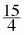3. 3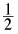4.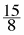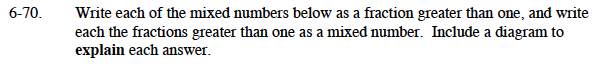Refer to the Math Notes box from Lesson 6.2.2.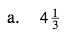This can be re-written as:

$\frac{3}{3}+\frac{3}{3}+\frac{3}{3}+\frac{3}{3}+\frac{1}{3}$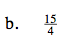This can be re-written as:

$\frac{4}{4}+\frac{4}{4}+\frac{4}{4}+\frac{3}{4}$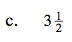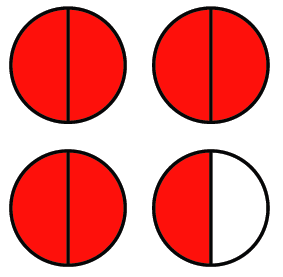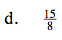$1\frac{7}{8}$